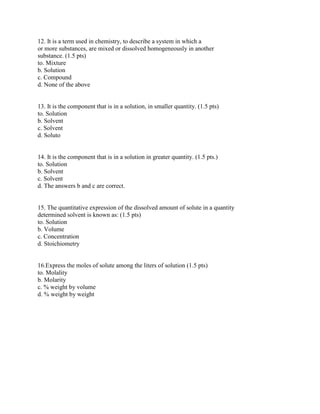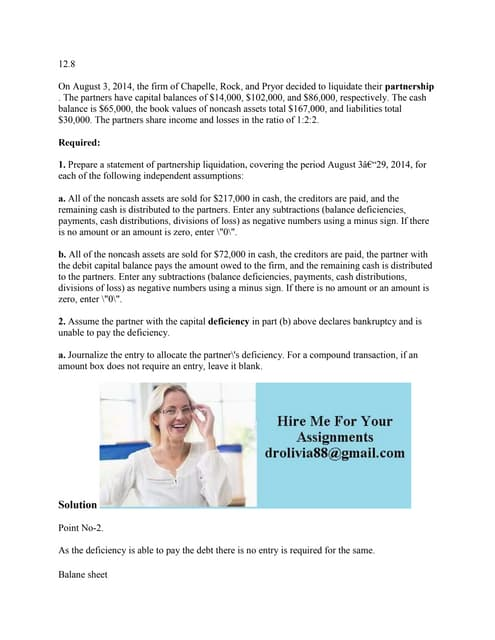Anúncio

# 12- It is a term used in chemistry- to describe a system in which a or.docx

todd991
8 de Feb de 2023Próximos SlideShares12-8 On August 3- 2014- the firm of Chapelle- Rock- and Pryor decided.docx
Carregando em ... 3
1 de 2
Anúncio

### 12- It is a term used in chemistry- to describe a system in which a or.docx

1. 12. It is a term used in chemistry, to describe a system in which a or more substances, are mixed or dissolved homogeneously in another substance. (1.5 pts) to. Mixture b. Solution c. Compound d. None of the above 13. It is the component that is in a solution, in smaller quantity. (1.5 pts) to. Solution b. Solvent c. Solvent d. Soluto 14. It is the component that is in a solution in greater quantity. (1.5 pts.) to. Solution b. Solvent c. Solvent d. The answers b and c are correct. 15. The quantitative expression of the dissolved amount of solute in a quantity determined solvent is known as: (1.5 pts) to. Solution b. Volume c. Concentration d. Stoichiometry 16.Express the moles of solute among the liters of solution (1.5 pts) to. Molality b. Molarity c. % weight by volume d. % weight by weight
2. Solution 12. The correct answer is B) Solution . It is a term used in chemistry to describe a system in which one or more substances are mixed or dissolved homogeneously in another substance. The given statement is definition of Solution 13. The correct option is D) Solute It is the component that is in a solution, in smaller quantity. 14. The correct option is D) The answers b and c are correct. It is the component that is in a solution in greater quantity. 15. The correct option is C) Concentration The quantitative expression of the dissolved amount of solute in a quantity determined solvent is known as: 16. The correct answer is B) Molarity Express the moles of solute among the liters of solution
Anúncio